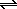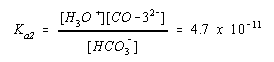Compounds That Could Be Either Acids or Bases

Compounds That Could Be Either Acids or Bases

Sometimes the hardest part of a calculation is deciding whether the compound is an acid or a base. Consider sodium bicarbonate, for example, which dissolves in water to give the bicarbonate ion.

 H2O NaHCO3(s)Na+(aq) + HCO3-(aq)

In theory, the bicarbonate ion can act as both a Brnsted acid and a Brnsted base toward water.

HCO3-(aq) + H2O(l)H3O+(aq) + CO32-(aq)

HCO3-(aq) + H2O(l)H2CO3(aq) + OH-(aq)

Which reaction predominates? Is the HCO3- ion more likely to act as an acid or as a base?

We can answer this question by comparing the equilibrium constants for these reactions. The equilibrium in which the HCO3- ion acts as a Brnsted acid is described by Ka2 for carbonic acid.The equilibrium in which the HCO3- acts as a Brnsted base is described by Kb2 for the carbonate ion.Since Kb2 is significantly larger than Ka2, the HCO3- ion is a stronger base than it is an acid.

 Practice Problem 8:Predict whether solutions of the H2PO4- and HPO42- ions are more likely to be acidic or basic. Phosphoric acid, H3PO4, is obviously an acid. The phosphate ion, PO43-, is a base. Click here to check your answer to Practice Problem 8